>>

 Math >> Arithmatic >> Mathematics >> Fraction
Login or Register   Fractions - Mathematical Operations of Fractions
 New User Register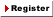Login Member: Email: Password:
 Site Search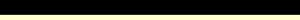Advance Search

Other animations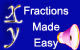Animation product List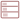Row formatGrid format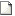List format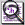Overview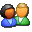(For age - group : Below 16 )

Fully animated ‘Mathematical Operations of Fractions’ simplifies the addition, subtraction, multiplication and division of fractions. It also elucidates the process of solving complex fractions problems into simpler using the BODMAS rule.Product - Animation
 Size/Time Subscribe Preview / Trailer Size (KB) 5803 US\$ 18.50    1.50 Rs. 108.00  Check price in your currency Subscription Days = 30 Watch a preview (opens in separate window) Time (hr:min:sec) 1:15:0 Add To CartPreview Unavailable

 This animation has audio.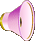Category : Math Fractions Type : Animation with sound Animation Type : Regular Total animation length: 75 minutes Watch combined preview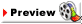The animation has the following broad sections: Adding Fractions Subtracting Fractions Dividing Fractions Multiplying Fractions Reciprocal of a fraction Reducing Fractions Divisibility Rules of fractions Simplifying complex fractions Reference Sections Check Your understanding Refer details section for the table of content.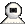Snapshots
 Watch combined preview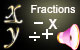Watch Fraction Made Easy or its other parts 1) Fundamental Concepts of Fractions 2) Kinds of Fractions 3) Mathematical Operations of Fractions shown above. Click on the above thumbnails to go to the fraction movie.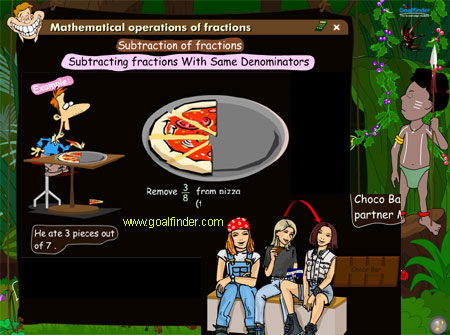This is an intuitive and interactive approach to fractions; there are short situational stories inside that explain the math concepts in a lucid manner inside a natural and real life environment.A systematic approach explains additions of fractions, subtraction of fractions, multiplication of fractions and division of fractions in a detailed style. An elaborate treatment of simplifying complex fractions clears the confusing math concept.This is like an animated math tutorial but with entertainment and stories to make learning fractions a fun.Details of the animation/ movie /software

Following topics are covered in a detailed animated manner.

• Adding fractions and subtracting fractions
• Adding fractions with the same denominator
• Adding fractions with different denominators
• Adding mixed fractions with the same denominator
• Subtract fractions with same denominators
• Subtract fractions with different denominators
• Adding and subtracting mixed numbers
• Multiplying fractions and whole numbers
• Multiplying fractions and fractions
• Multiplying mixed numbers
• Reciprocal of a fraction
• Dividing fractions
• Dividing mixed fractions
• Simplifying complex fractions
• Greatest common factor
• Least common multiple
• Equivalent fractions
• Converting and reducing fractions
• Lowest terms in fractions
• Check your understanding section after chapters.Related Products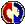Buyers Feedback: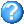Q & A
 Q1: how to use the four mathematical operation in fraction? erwin demen 22/6/2010 Ans:Give your comments